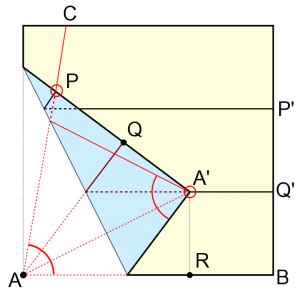There are some problems that trouble so many people for so long that they become ‘classics’. Things like Fermat’s Last Theorem (which lasted 350-odd years without a proof), how to factorise big numbers (still waiting) and proving the parallel postulate from the other Euclidean axioms (about 2,300 years before people realised it can’t be done).

A classical question from the same era was this: given a compass and a straight-edge, the only tools a mathematician would ever carry, can you divide an angle into three equal parts?

The answer, it turns out, is no - Pierre Wantzel showed it was impossible in 1837, using some ideas from Galois. However, if that was the end of the story, it’d be a very dull story.

It turns out you can trisect an angle on a piece of paper ((in fairness to Euclid, paper hadn’t been invented)) by folding it cleverly, like this: [caption id=”attachment_2505” align=”alignright” width=”300”]Trisecting an angle using origami (via Wikipedia)[/caption]

This was discovered by Hisashi Abe. You start with angle AC marked, and make a horizontal fold (PP’). You then make a second fold (QQ’) midway between AB and PP’ (by folding AB up to meet PP’).

Here’s the clever bit: you now need to make a fold that makes point P lie on the original AC line, and point A on the line QQ’ (becoming point A’). It’s a bit tricky to get this right, but totally possible. Now, triangles APQ and AA’Q are congruent - they’re right-angled, they share the side AQ and PQ = A’Q because Q is midway between them. They’re also congruent to the triangle AA’R, because it is right-angled, shares the side AA’ with AA’Q and A’R = A’Q.

That means the three angles at A are equal and add up to CAB, meaning we’ve trisected the angle - go us!

It turns out that the maths of origami is a bit more flexible((no pun intended. Well, ok, maybe a little one.)) than the maths of straight-edges and compasses: origami allows you to solve cubic equations, while classical geometry only lets you loose on quadratics.

And origami’s not just an abstract thing, either: the Miura fold, for instance, allows solar panels to be folded up in one smooth motion; vehicle designers now use the principles of origami to figure out how car bodies should behave in a collision.

Plus, the really important bit: you can use origami to make swans and stuff.